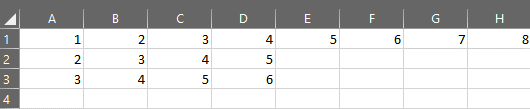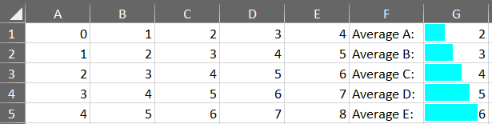With the latest release, R2 2022, we added support for using references to whole columns and rows for the SpreadProcessing library. Let’s take a closer look.

Formulas are one of the most powerful tools that a spreadsheet provides. When referencing the required range for a formula, a specific cell reference often proves less convenient than we would like. This is where this new feature comes in to save the day.

Suppose we want to sum all numbers in the first row of the following example worksheet:As you can see, the first row contains numbers up to column H. We could use a standard cell reference in the formulas such as =SUM(A1:H1). Although this would work for the time being, the worksheet’s data might undergo changes in the future, which could affect the formula and render it erroneous.

With the addition of the whole column/row references, we can easily bypass such potential issues. Here is how the above formula would look using a whole row reference: =SUM(1:1). You can set a cell’s value using the newly supported references just as you would using the cell references. In the below code, we create a new workbook, add a new worksheet to it, and then set the value of cell B1 to the above formula:

``````Workbook workbook = new Workbook();

worksheet.Cells[1,0].SetValue("=SUM(1:1)"); ``````

The same applies to columns. Alongside referencing specific cells, you can now use entire column references. In the worksheet above, the formula =SUM(A1:D3), would return the same result as =SUM(A:D).

## Retaining High Performance

Referencing entire rows and columns could mean including thousands or even millions of cells in a cell range, which could impact a worksheet’s performance. Therefore, our team has taken care to maintain the library’s high efficiency when implementing the feature. Not sure? Try it yourself and be pleasantly surprised with how blazingly fast formulas evaluate.

## Practical Application

When introducing a new tool, it is always easier to understand when and how to use it by sharing a practical use case scenario where it comes in handy. Since our team recently implemented one of the most requested features, Conditional Formatting, we can use it as a context for showing off some benefits of referencing entire rows and columns. Conditional Formatting helps us analyze data visually by configuring a set of formatting rules to be applied over a specified range.

In the code below, we create a small table in an existing Worksheet, then append a row on its right, which shows the average for each column. Instead of referencing the beginning and end cells of each column in the formula used, we can easily reference the entire columns:

``````var tableSize = 5;
for (int row = 0; row < tableSize; row++)
{
for (int col = 0; col < tableSize; col++)
{
worksheet.Cells[row, col].SetValue(row + col);
}
}
char asciiColSymbol = 'A';
for (int row = 0; row < tableSize ; row++)
{
string headerColumnText = string.Format("Average {0}:", asciiColSymbol);
string formula = string.Format("=AVERAGE({0}:{1})", asciiColSymbol, asciiColSymbol);
worksheet.Cells[row, tableSize+1].SetValue(formula);

asciiColSymbol++;
}

DataBarValueContext dataBarValueContext = new DataBarValueContext();
DataBarRule dataBarRule = new DataBarRule(dataBarValueContext);
dataBarRule.FillColor = new ThemableColor(Colors.Aqua);
ConditionalFormatting conditionalFormatting = new ConditionalFormatting(dataBarRule);

var averageColumn=tableSize+1;
``````

Here we can see the exported worksheet with the column averages:Don’t forget to check out our new Conditional Formatting feature in more detail in the Conditional Formatting blog article.

If you have yet to try Telerik Document Processing, you can download a free trial and check out the fantastic features the libraries support.

If you are already using the libraries, we are always eager to receive your feedback. Don’t be shy and drop us a comment with your thoughts or visit our Document Processing Libraries Feedback Portal and let us know if you have any suggestions or need any specific functionality implemented.### Svilen Pavlov

Svilen is a Tech Support Engineer in the Document Processing team. His main responsibility is assisting clients with using the team's libraries and implementing them in their work. He spends his free time travelling, playing sports, board games and attending music festivals.